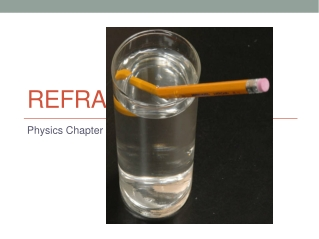DownloadDownload PresentationRefraction

# Refraction

Download Presentation## Refraction

- - - - - - - - - - - - - - - - - - - - - - - - - - - E N D - - - - - - - - - - - - - - - - - - - - - - - - - - -
##### Presentation Transcript

1. Refraction Physics Chapter 18b

2. Refraction • Bending rays of light as it passes from one medium to another • Caused by change in speed of wave • Amount of refraction depends on angle of incidence and properties of the two media • Optical density • Property of medium that determines speed of light in that medium

3. Snell's Law • Each medium has a characteristic change in the speed of the wave • This characteristic is called the index of refraction • Amount of bending depends on the index of refraction • For a wave travelling from a vacuum into another medium: • n = sin i • sin r

4. TABLE OF INDEX OF REFRACTION VALUES • (For Sodium yellow light): • Material INDEX OF REFRACTION: n=c/v • Water 1.33 • Fused quartz 1.46 • Crown glass 1.52 • Lucite or Plexiglass 1.51 • Diamond 2.42 • Ethyl Alcohol 1.36 • Air (at STP) 1.0003 • Light flint 1.58 • Sodium chloride 1.53

5. Snell's Law for Any Two Media • Index of refraction of first medium times the sine of the angle of incidence equals the index of refraction of the second medium times the sine of the angle of refraction • Both angles measured from normal

6. Example • A light beam in air hits a sheet of crown glass at an angle of 30o. At what angle is the beam refracted? • What we know: incident angle 30o incident n: 1.0003 refracting n: 1.52 • Equation: n1sinΘ1 = n2sinΘ2 • Put values in: (1.0003)sin30 = (1.52)sinΘ

7. Example Continued • (1.0003)(.5) = 1.52sinΘ • 0.50015/1.52 = sinΘ • 0.329046053 = sinΘ • Θ = 19.210885 = 19.2o